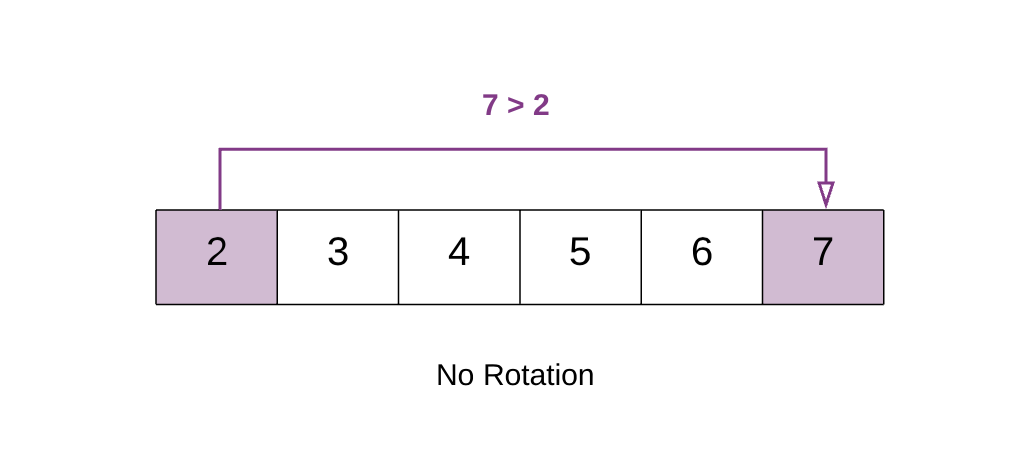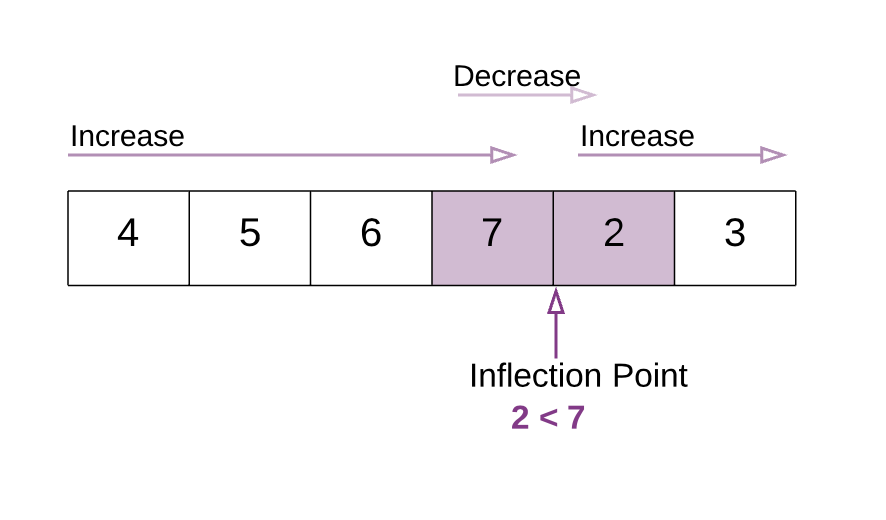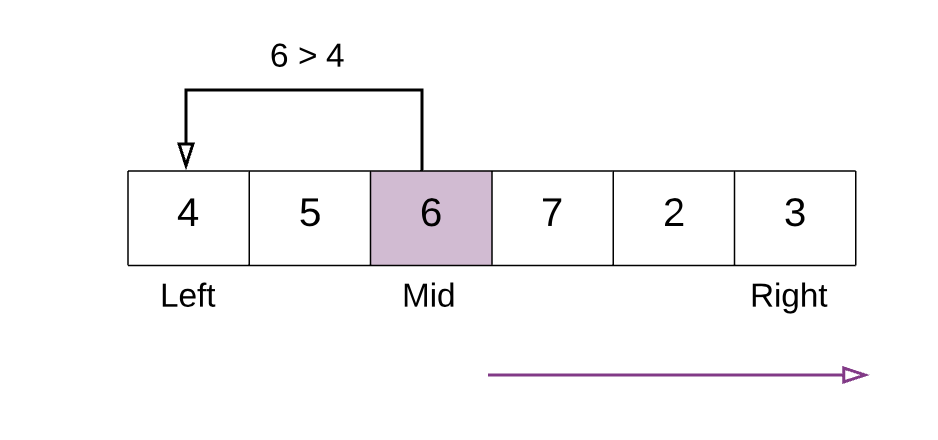## Solution

Intuition

A very brute way of solving this question is to search the entire array and find the minimum element. The time complexity for that would be given that N is the size of the array.

A very cool way of solving this problem is using the Binary Search algorithm. In binary search we find out the mid point and decide to either search on the left or right depending on some condition.

Since the given array is sorted, we can make use of binary search. However, the array is rotated. So simply applying the binary search won't work here.

In this question we would essentially apply a modified version of binary search where the condition that decides the search direction would be different than in a standard binary search.

We want to find the smallest element in a rotated sorted array. What if the array is not rotated? How do we check that?

If the array is not rotated and the array is in ascending order, then last element > first element.In the above example 7 > 2. This means that the array is still sorted and has no rotation.In the above example 3 < 4. Hence the array is rotated. This happens because the array was initially [2, 3 ,4 ,5 ,6 ,7]. But after the rotation the smaller elements[2,3] go at the back. i.e. [4, 5, 6, 7, 2, 3]. Because of this the first element  in the rotated array becomes greater than the last element.

This means there is a point in the array at which you would notice a change. This is the point which would help us in this question. We call this the Inflection Point.In this modified version of binary search algorithm, we are looking for this point. In the above example notice the Inflection Point .

All the elements to the left of inflection point > first element of the array.
All the elements to the right of inflection point < first element of the array.

Algorithm

1. Find the mid element of the array.

2. If mid element > first element of array this means that we need to look for the inflection point on the right of mid.

3. If mid element < first element of array this that we need to look for the inflection point on the left of mid.In the above example mid element 6 is greater than first element 4. Hence we continue our search for the inflection point to the right of mid.

4 . We stop our search when we find the inflection point, when either of the two conditions is satisfied:

nums[mid] > nums[mid + 1] Hence, mid+1 is the smallest.

nums[mid - 1] > nums[mid] Hence, mid is the smallest.In the above example. With the marked left and right pointers. The mid element is 2. The element just before 2 is 7 and 7>2 i.e. nums[mid - 1] > nums[mid]. Thus we have found the point of inflection and 2 is the smallest element.

Complexity Analysis

• Time Complexity : Same as Binary Search
• Space Complexity :

Analysis written by: @godayaldivya.